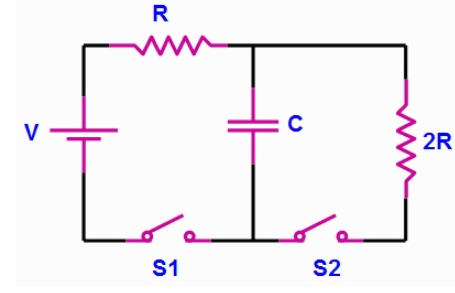# Problem: A circuit is wired up as shown below. The capacitor is initially uncharged and switches S1 and S2 are initially open.1) What is the voltage across the capacitor immediately after switch S1 is closed?a) Vc = 0b) Vc = Vc) Vc = 2V/32) What is the voltage across the capacitor after switch S1 has been closed for a very long time?a) Vc = 0b) Vc = Vc) Vc = 2V/33) After being closed a long time, switch 1 is opened and switch 2 is closed. What is the current through the right resistor immediately after switch 2 is closed?a) IR = 0b) IR = V/3Rc) IR = V/2Rd) IR = V/R4) Now suppose both switches are closed. What is the voltage across the capacitor after a very long time?a) Vc = 0b) Vc = Vc) Vc = 2V/3

###### FREE Expert Solution

1)

Voltage;

$\overline{){\mathbf{V}}{\mathbf{=}}\frac{\mathbf{Q}}{\mathbf{C}}}$

95% (46 ratings)###### Problem Details

A circuit is wired up as shown below. The capacitor is initially uncharged and switches S1 and S2 are initially open.1) What is the voltage across the capacitor immediately after switch S1 is closed?

a) Vc = 0

b) Vc = V

c) Vc = 2V/3

2) What is the voltage across the capacitor after switch S1 has been closed for a very long time?

a) Vc = 0

b) Vc = V

c) Vc = 2V/3

3) After being closed a long time, switch 1 is opened and switch 2 is closed. What is the current through the right resistor immediately after switch 2 is closed?

a) IR = 0

b) IR = V/3R

c) IR = V/2R

d) IR = V/R

4) Now suppose both switches are closed. What is the voltage across the capacitor after a very long time?

a) Vc = 0

b) Vc = V

c) Vc = 2V/3

Frequently Asked Questions

What scientific concept do you need to know in order to solve this problem?

Our tutors have indicated that to solve this problem you will need to apply the !! Resistor-Capacitor Circuits concept. If you need more !! Resistor-Capacitor Circuits practice, you can also practice !! Resistor-Capacitor Circuits practice problems.

What professor is this problem relevant for?

Based on our data, we think this problem is relevant for Professor Mannino's class at University of New Haven.